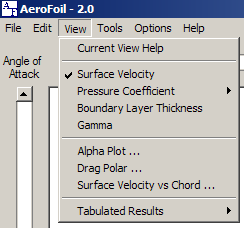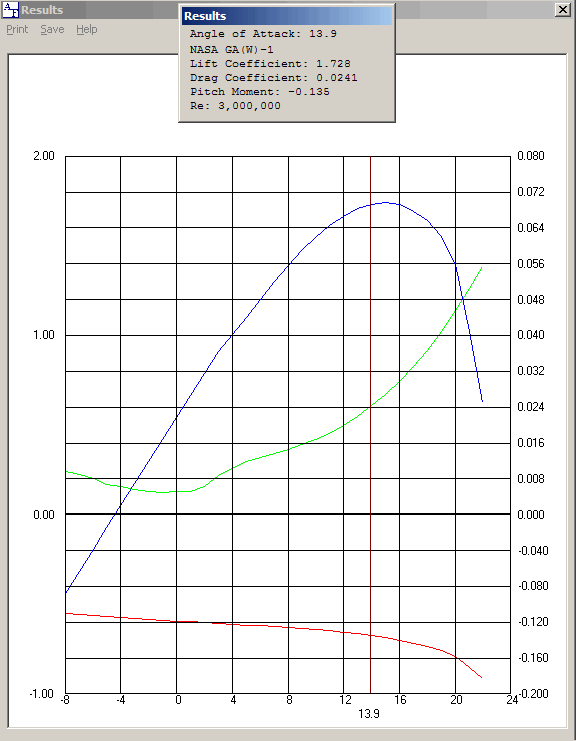## Alpha Plot - Lift, Drag, and Pitch vs Angle of AttackThe lift coefficient is displayed in blue, the Pitch Coefficient in red, and if the Viscous option is selected, the drag coefficient in green.

The Pitch Coefficient is about the airfoil's quarter chord location, x/c = 0.25 and y/c = 0.0.

In the case of inviscid calculations, the lift coefficient and Pitch Coefficient are approximately linear.The left vertical axis is the lift coefficient. The lower right vertical axis is the Pitch Coefficient and the upper right vertical axis is the drag coefficient. The horizontal axis is the angle of attack in degrees.

Calculated results that are significantly after the point of stall may not be valid. In this case, the angle of attack used for the calculations may be adjusted as necessary.

Values at discreet angles of attack may be examined by clicking the left mouse button and dragging the angle of attack line left or right.